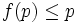# Intermediately operator

This article defines a subgroup property modifier (a unary subgroup property operator) -- viz an operator that takes as input a subgroup property and outputs a subgroup property

View a complete list of subgroup property modifiers OR View a list of all subgroup property operators (possibly with multiple inputs)

This property modifier is idempotent and a property is a fixed-point, or equivalently, an image of this if and only if it is a:intermediate subgroup condition

## Definition

### Symbol-free definition

The intermediately operator is a map from the subgroup property space to itself, that sends a subgroup property$p$ to the property of being a subgroup that satisfies$p$ not only in the whole group, but also in every intermediate subgroup.

### Definition with symbols

Given a subgroup property$p$, the subgroup property intermediately$p$ is the property as follows:$H$ satisfies intermediately$p$ in$G$ if for any group$K$ with$H \le K \le G$,$H$ satisfies$p$ in$K$.

## Properties

### Monotonicity

This subgroup property modifier is monotone, viz if$p \le q$ are subgroup properties and$f$ is the operator, then$f(p) \le f(q)$

If$p \le q$ are two subgroup properties, then intermediately$p \le$ intermediately$q$. This follows directly from the definition.

### Descendance

This subgroup property modifier is descendant, viz the image of any subgroup property under this modifier is stronger than that property. In symbols, if$f$ denotes the modifier and$p$ and property,$f(p) \le p$ For any subgroup property$p$, intermediately$p \le p$. This follows from the fact that if$H$ satisfies property$p$ in every intermediate subgroup,$H$ also satisfies property$p$ in the whole group.

### Idempotence

This subgroup property modifier is idempotent, viz applying it twice to a subgroup property has the same effect as applying it once

The intermediately operator is idempotent, in the sense that applying the intermedaitely operator twice has the same effect as applying it once. The image-cum-fixed-point-space for this operator is precisely the subgroup properties satisfying the intermediate subgroup condition.

## Effect on metaproperties

Suppose$p$ is a join-closed subgroup property, viz the join of any family of subgroups satisfying property$p$, also satisfies property$p$. Then, it is easy to see that intermediately$p$ is also join-closed.

### Transitivity

It is not clear whether, even if$p$ is transitive, intermediately$p$ will b transitive.

### Transfer condition

If$p$ satisfies the transfer condition, it also, in particular, satisfies the intermediate subgroup condition, and hence$p$ is unchanged under application of the intermediately operator.

These include: